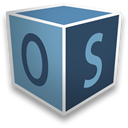OpenStudio:utilities
openstudio::Transformation Class Reference

#include <Transformation.hpp>

Public Member Functions

EulerAngles eulerAngles () const
get the Euler angles for the transformation, does not include translation More...

Transformation inverse () const
returns a transformation which is the inverse of this More...

Matrix matrix () const
get the matrix representation directly More...

Point3d operator* (const Point3d &point) const
apply the transformation to the point More...

Vector3d operator* (const Vector3d &vector) const
apply the transformation to the vector More...

BoundingBox operator* (const BoundingBox &boundingBox) const
apply the transformation to the bounding box More...

Plane operator* (const Plane &plane) const
apply the transformation to the plane More...

std::vector< Point3doperator* (const std::vector< Point3d > &points) const
apply the transformation to a vector of points More...

std::vector< Vector3doperator* (const std::vector< Vector3d > &vectors) const
apply the transformation to a vector of vector More...

Transformation operator* (const Transformation &other) const
apply the transformation to the other transformation More...

Matrix rotationMatrix () const
get the rotation matrix for the transformation, does not include translation More...

Transformation ()
default constructor creates identity transformation More...

Transformation (const Matrix &matrix)
constructor from storage, asserts matrix is 4x4 More...

Transformation (const Vector &vector)
constructor from storage, asserts vector is size 16 More...

Transformation (const Transformation &other)
copy constructor More...

Vector3d translation () const
get the translation for the transformation, does not include rotation More...

Vector vector () const
get the vector representation directly More...

Static Public Member Functions

static Transformation alignFace (const std::vector< Point3d > &vertices)
transforms face coordinates to regular system, face normal will be z' will try to align y' with z, but if that fails will align y' with y face origin will be minimum point in x', y' and z'=0 will return identity transformation if cannot compute plane for vertices More...

static Transformation alignZPrime (const Vector3d &zPrime)
transforms system with z' to regular system will try to align y' with z, but if that fails will align y' with y More...

static Transformation rotation (const Vector3d &axis, double radians)

static Transformation rotation (const Point3d &origin, const Vector3d &axis, double radians)

static Transformation rotation (const EulerAngles &angles)
rotation specified by Euler angles More...

static Transformation translation (const Vector3d &translation)
translation along vector More...

Constructor & Destructor Documentation

 openstudio::Transformation::Transformation ( )

default constructor creates identity transformation

 openstudio::Transformation::Transformation ( const Matrix & matrix )

constructor from storage, asserts matrix is 4x4

 openstudio::Transformation::Transformation ( const Vector & vector )

constructor from storage, asserts vector is size 16

 openstudio::Transformation::Transformation ( const Transformation & other )

copy constructor

Member Function Documentation

 static Transformation openstudio::Transformation::alignFace ( const std::vector< Point3d > & vertices )
static

transforms face coordinates to regular system, face normal will be z' will try to align y' with z, but if that fails will align y' with y face origin will be minimum point in x', y' and z'=0 will return identity transformation if cannot compute plane for vertices

 static Transformation openstudio::Transformation::alignZPrime ( const Vector3d & zPrime )
static

transforms system with z' to regular system will try to align y' with z, but if that fails will align y' with y

 EulerAngles openstudio::Transformation::eulerAngles ( ) const

get the Euler angles for the transformation, does not include translation

 Transformation openstudio::Transformation::inverse ( ) const

returns a transformation which is the inverse of this

 Matrix openstudio::Transformation::matrix ( ) const

get the matrix representation directly

 Point3d openstudio::Transformation::operator* ( const Point3d & point ) const

apply the transformation to the point

 Vector3d openstudio::Transformation::operator* ( const Vector3d & vector ) const

apply the transformation to the vector

 BoundingBox openstudio::Transformation::operator* ( const BoundingBox & boundingBox ) const

apply the transformation to the bounding box

 Plane openstudio::Transformation::operator* ( const Plane & plane ) const

apply the transformation to the plane

 std::vector openstudio::Transformation::operator* ( const std::vector< Point3d > & points ) const

apply the transformation to a vector of points

 std::vector openstudio::Transformation::operator* ( const std::vector< Vector3d > & vectors ) const

apply the transformation to a vector of vector

 Transformation openstudio::Transformation::operator* ( const Transformation & other ) const

apply the transformation to the other transformation

 static Transformation openstudio::Transformation::rotation ( const Vector3d & axis, double radians )
static

 static Transformation openstudio::Transformation::rotation ( const Point3d & origin, const Vector3d & axis, double radians )
static

 static Transformation openstudio::Transformation::rotation ( const EulerAngles & angles )
static

rotation specified by Euler angles

 Matrix openstudio::Transformation::rotationMatrix ( ) const

get the rotation matrix for the transformation, does not include translation

 static Transformation openstudio::Transformation::translation ( const Vector3d & translation )
static

translation along vector

 Vector3d openstudio::Transformation::translation ( ) const

get the translation for the transformation, does not include rotation

 Vector openstudio::Transformation::vector ( ) const

get the vector representation directly